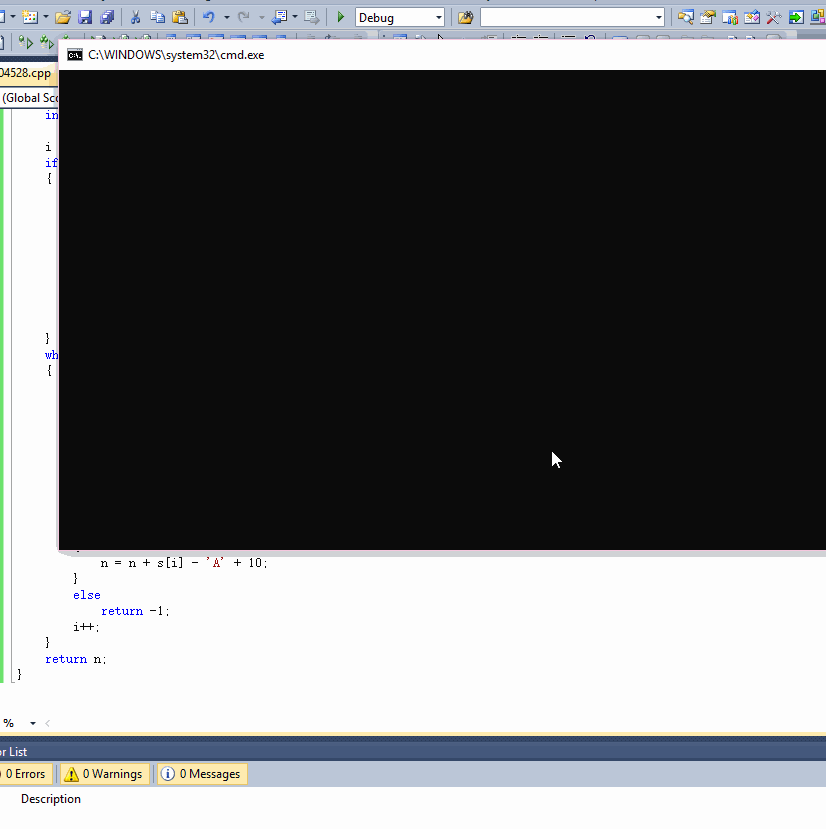C语言 显示字符串中的字符无效，调试了也不清楚哪儿的问题
`````` #include<stdio.h>

#define MAX 20//定义最大长度

//测试函数htoi
int htoi(char s[]);

int main()
{
int i = 0;
int n;
int c;
char s[MAX];

while ((c = getchar()) != EOF && c != '\n')
{
s[i] = c;
++i;
}
i++;
s[i] = '\0';
n = htoi(s);
printf("%d", n);
}

/*

*/
int htoi(char s[])
{
int i;
int n = 0;//要输出的整形值
int state;//判断字符是否为16进制

state = 1;//默认字符是十六进制
i = 0;
if (s[i] == '0')
{
i++;
if (s[i] == 'x' || s[i] == 'X')
i++;
}
while (s[i] != '\0'&&state != 0);
{
if (s[i] >= '0'&&s[i] <= '9')
{
n = n + s[i] - '\0';
i++;
}
else if (s[i] >= 'a'&&s[i] <= 'f')
{
n = n + s[i] - 'a' + 10;
i++;
}
else if (s[i] >= 'A'&&s[i] <= 'F')
{
n = n + s[i] - 'A' + 10;
i++;
}
else
state = 0;
}
if (state == 1)
return n;
else
return -1;
}
``````

4个回答

ERROR 1：
main函数中输入字符串结尾加\0的位置有问题，i不需要+1了

`````` while ((c = getchar()) != EOF && c != '\n')
{
s[i] = c;
++i;
}
s[i] = '\0';
``````

ERROR 2：
htoi函数中的while后面的分号去掉

``````while (s[i] != '\0'&&state != 0)
``````

ERROR 3：

`````` if (s[i] >= '0'&&s[i] <= '9')
{
n = n + s[i] - '0';
i++;
}
``````

`````` int htoi(char s[])
{
int i;
int n = 0;//要输出的整形值
int state;//判断字符是否为16进制
state = 1;//默认字符是十六进制
i = 0;
if (s[i] == '0')
{
i++;
if (s[i] == 'x' || s[i] == 'X')
i++;
}
while (s[i] != '\0'&&state != 0)
{
if (s[i] >= '0'&&s[i] <= '9')
{
n = n*16 + s[i] - '0';
}
else if (s[i] >= 'a'&&s[i] <= 'f')
{
n = n*16 + s[i] - 'a' + 10;
}
else if (s[i] >= 'A'&&s[i] <= 'F')
{
n = n*16 + s[i] - 'A' + 10;
}
else
state = 0;
i++;
}
if (state == 1)
return n;
else
return -1;
}
``````

`````` // Q704528.cpp : Defines the entry point for the console application.
//

#include "stdafx.h"

#include<stdio.h>

#define MAX 20//定义最大长度

//测试函数htoi
int htoi(char s[]);

int main()
{
int i = 0;
int n;
int c;
char s[MAX];

while ((c = getchar()) != EOF && c != '\n')
{
s[i] = c;
++i;
}
s[i] = '\0';
n = htoi(s);
printf("%d\n", n);
}

/*

*/
int htoi(char s[])
{
int i;
int n = 0;//要输出的整形值
//int state;//判断字符是否为16进制

i = 0;
if (s[i] == '0')
{
i++;
if (s[i] == 'x' || s[i] == 'X')
{
i++;
}
else
{
i--;
}
}
while (s[i] != '\0')
{
n *= 16;
if (s[i] >= '0'&&s[i] <= '9')
{
n = n + s[i] - '0';
}
else if (s[i] >= 'a'&&s[i] <= 'f')
{
n = n + s[i] - 'a' + 10;
}
else if (s[i] >= 'A'&&s[i] <= 'F')
{
n = n + s[i] - 'A' + 10;
}
else
return -1;
i++;
}
return n;
}

```````如果问题得到解决，麻烦点下我回答右边的采纳，谢谢`

`````` #include<stdio.h>

#define MAX 20//定义最大长度

//测试函数htoi
int htoi(char s[]);

int main()
{
int i = 0;
int n;
int c;
char s[MAX];

while ((c = getchar()) != EOF && c != '\n')//这一段循环输入字符串，可以用gets(s)函数，或者scanf("%s",s)
{
s[i] = c;
++i;
}
//i++;改：此处不能增加i,在循环中已经增加过一次了
s[i] = '\0';
n = htoi(s);
printf("%d\n", n);
}

/*

*/
int htoi(char s[])
{
int i;
int n = 0;//要输出的整形值
int state;//判断字符是否为16进制

//state = 1;//默认字符是十六进制----改：默认应该为零，如果按照你以下的写法
state = 0;//后面也用不到这个
i = 0;
if (s[i] == '0')
{
i++;
if (s[i] == 'x' || s[i] == 'X')state = 1;//改：此处确认输入是否为16进制
else return -1;
i++;
}
else return -1;//改：如果不是，直接返回-1
/*以下的做法完全是错的，没办法改了，想打个补丁都不行
while (s[i] != '\0'&&state != 0);
{
if (s[i] >= '0'&&s[i] <= '9')
{
//n = n + s[i] - '\0';   '\0’就是十进制的0，这个减法没有任何意义。16进制转换也不是这么算的
i++;
}
else if (s[i] >= 'a'&&s[i] <= 'f')
{
n = n + s[i] - 'a' + 10;
i++;
}
else if (s[i] >= 'A'&&s[i] <= 'F')
{
n = n + s[i] - 'A' + 10;
i++;
}
else
state = 0;
}
if (state == 1)
return n;
else
return -1;*/

//改：
//字符串的方向与数值得方向刚好相反，字符串是低位在左，高位在右。数值是低位在右，高位在左
//所以读取16进制字符串应该能从右边开始

int j = i;
while (s[j]!='\0')
{
j++;//将索引至于字符串s的最右边
}
j--;//后退到‘\0’前一位

int r = 1;//16进制位权，初始化为1
while (j>=i)
{
if (s[j] >= '0'&&s[j] <= '9')
{
n = n + r*(s[j] - '0');
}
else if(s[j] >= 'A'&&s[j] <= 'F')
{
n = n + r*(s[j] - 'A' + 10);
}
else if (s[j] >= 'a'&&s[j] <= 'f')
{
n = n + r*(s[j] - 'a' + 10);
}
else return -1;//如果包含其他非法字符，返回-1

r = r * 16;//增加位权
j--;

}

return n;

}
``````